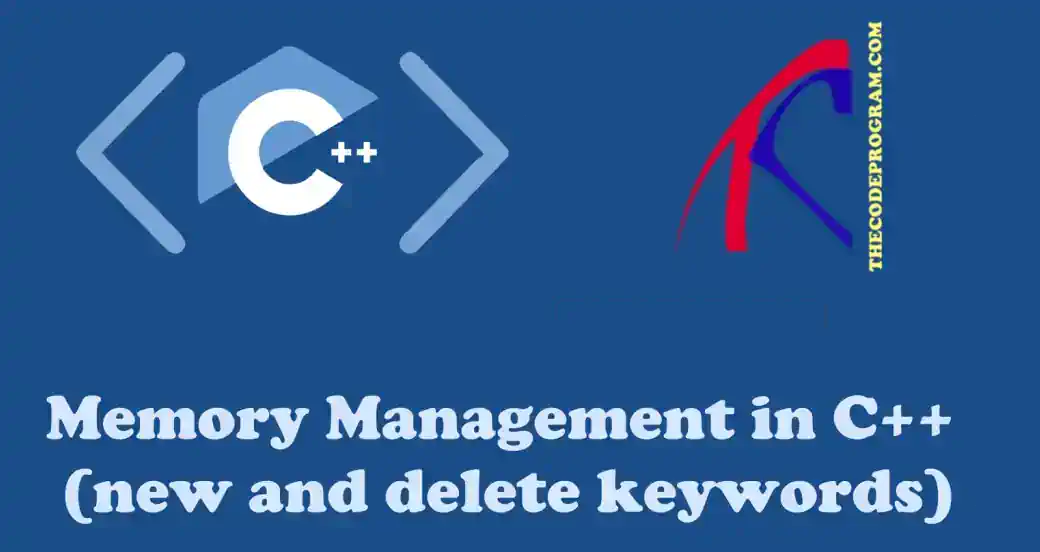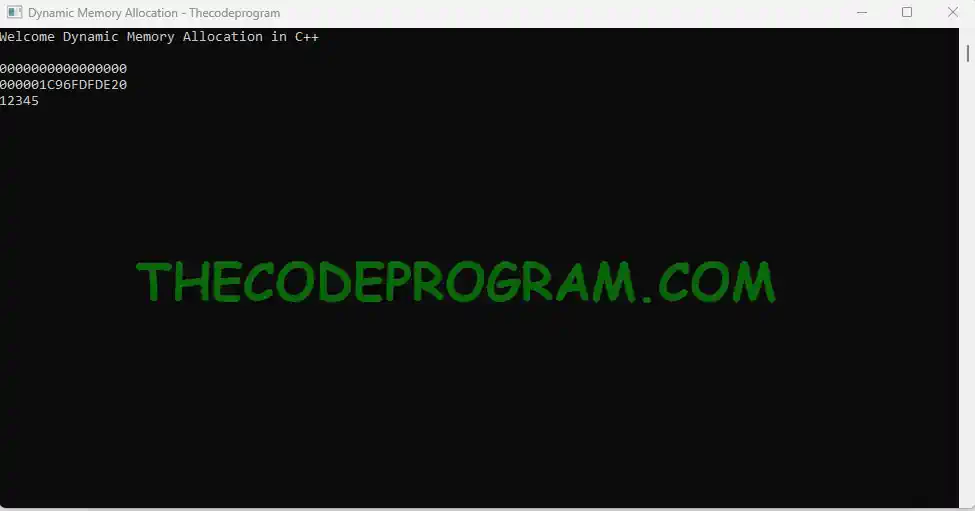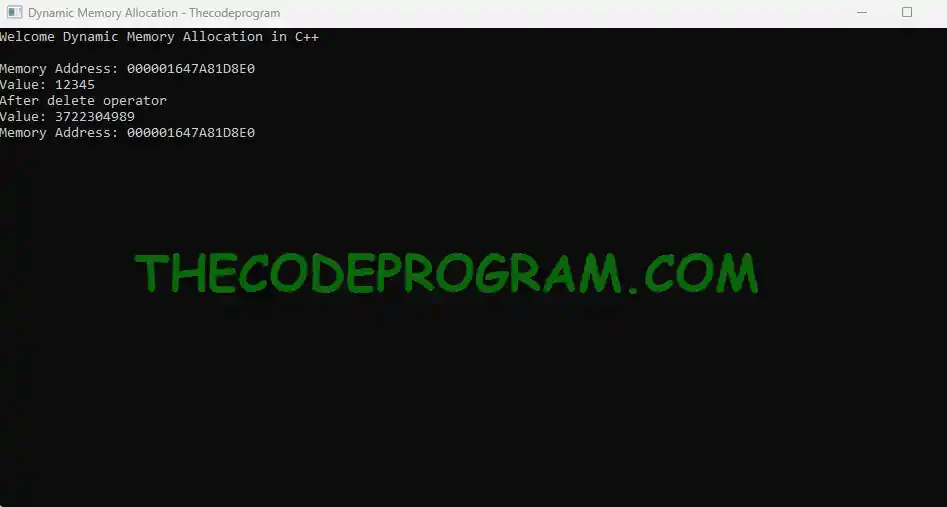## Memory Management in C++ (new and delete keywords)

Hello everyone, in this article we are going to talk about new and delete keywords in C++. We will talk about the dynamic memory management programmatically in C++.

Let's begin.

In most of new generatıion programming languages memory management process handling automatically. In C++ we manage the memory and allocate the data in memory. In C++ ıt ıs called as dynamic memory allocation.

In C++ we allocate new memory wıth initializing a new variable and we can set free memory after they are used.
• For allocation we use new keyword
• For deallocation we use delete keyword
Below you can see a simple syntax and example about allocating memory for a variable
``````
//Declaring a variable
uint32_t* data;
cout << data << endl; //Print 1

//Allocating memory for variable
data = new uint32_t;
cout << data << endl; //Print 2

//Assigning a value
*data = 12345;
cout << *data << endl; //Print 3
``````
Below image you can see output of above exampleAs you can see we have allocated the variable dynamically. At first and second steps we have printed the memory addresses of the variable
• At first step we declared a variable and and we printed memory location of variable. It is not allocated yet and memory location is "0" at Print 1
• At second step variable is initialized with "new" keyword it is allocated and we can see the memory location in Print 2
• At third step we assigned a value to value and we can see the value of that variable at Print 3

Until here we allocated memory for the variable, now after we don't need anymore we need to deallocate the memory and set free. For deallocation the memory we use delete operator. With delete operator we remove the assignee from memory and we set free the memory from the variable.

When we print the deleted variable we see the 0xDDDDDDDD (3722304989) magic number which specified by Microsoft for released memory heap. So lets make an example about usage of delete keyword. (About magic numbers for you can check wikipedia Programming magic Numbers Debug Values by Microsoft

Below you can see the basic syntax and usage of delete operator, First part is same with that we did above, after it we just added the delete operator section
``````
//Declaring a variable
uint32_t* data = new uint32_t;
cout << "Memory Address: " << data << endl;
//Assigning a value
*data = 12345;
cout << "Value: " << *data << endl;

// Delete the variable
delete data;
cout << "After delete operator" << endl;
cout << "Value: " << *data << endl; //Print 4
cout << "Memory Address: " << data << endl; //Print 5
``````
Program output will be like belowAs you can see above we allocated the memory dynamically with the delete operator

delete operator is important for the applications, If we dont remove the not needed variables from the memory, they will stay in the memory and may cause lots of system resource usage. So to reduce memory usage we need to delete the variables and set free the memory if we don't need anymore.

For demonstrating the importance of delete operator lets make a simple application. Below example has written by qt. Lets run below application without delete operator in for loop first and then uncomment the delete operator and run again.
``````
#include <iostream>
using namespace std;
//QT Library
#include <QCoreApplication>
int main(int argc, char *argv[])
{
QCoreApplication a(argc, argv);

system("title Dynamic Memory Allocation - Thecodeprogram");
cout << "Welcome Dynamic Memory Allocation in C++" << endl << endl;
for(long i=0; i<999999999; i++){
long* data = new long;
*data = i;
cout << "Value: " << *data << endl;
//delete data;
}
return a.exec();
}
``````
##### Results of tests:

At first run we are not deleting the variables from memory and they are staying in the memory until application closes. So when we follow the application from resource manager we observe memory usage is always increasing.
At second run we are runnıng the application with delete operator and we are removing the variable from memory and setting free the memory location. At second run, we observe the memory usage is always around 1,1MB and was not increasing.

I wish everyone good memory management.

Burak Hamdi TUFAN

04/12/2015

28/07/2020

29/10/2020